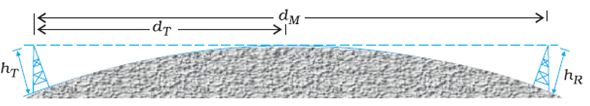# Communications (Test 2)

## Gate Exam : Ec Electronics And Communication Engineering

| Home | | Gate Exam | | Ec Electronics And Communication Engineering | | Communications |Communications
| Communications | | Communication Systems |
Q.1
The three elements of a generalized communication system are
A. Transmitter, transmission channel and receiver
C. Transmitter, noise generator and receiver
D. Transmitter, transmission channel and detector
Explaination / Solution:

In communication the Transmitter helps in transmitting the signal through communication channel which acts as a physical path that connects transmitter to a receiver.And the receiver receives the transmitted signal and converts those signals in their original form.

Workspace
Report
Q.2
In Analog type signals
A. the signal value is available every 1 second
B. the value of the signal varies continuously with time
C. the voltages are fixed at 0 and 5 V
D. the voltages are fixed at -5 V and 5 V
Explaination / Solution:

A signal in which current or voltage varies with time is called analog signal.A vibrating fork produces such a sound signal of single frequency.Every speech or music is a complex signal formed by the superposition of several sinusoidal signals of different amplitudes and frequencies.

Workspace
Report
Q.3
Modulation is
A. superimposing high frequency message on a low frequency wave carrier
B. superimposing low frequency message on a high frequency wave carrier
C. alternating low frequency message and a high frequency wave carrier
D. alternating high frequency message and a low frequency wave carrier
Explaination / Solution:

Modulation is the process of varying one or more properties of a periodic waveform, called the carrier signal, with a modulating signal that typically contains information to be transmitted. Most radio systems uses frequency modulation (FM) or amplitude modulation (AM) to make the carrier carry the radio broadcast.

Workspace
Report
Q.4
Audio signals have a maximum bandwidth of about
A. 2000 kHz
B. 2 kHz
C. 200 kHz
D. 20 kHz
Explaination / Solution:
No Explaination.

Workspace
Report
Q.5
With reference to the figure the value of  can be calculated byA.  dM=2RhT
B. dM=2RhR
C.  dM=2RhT+2RhR
D.  dM=2RhT+RhR
Explaination / Solution:
No Explaination.

Workspace
Report
Q.6
If S(f) is the power spectral density of a real, wide-sense stationary random process, then which of the following is ALWAYS true?
A. S(0)≤S(f)
B. S(f)≥0
C. S(-f) = -S(f)
D.Explaination / Solution:
No Explaination.

Workspace
Report
Q.7
The parallel branches of a 2-wire transmission line re terminated in 100Ω and 200Ω resistors as shown in the figure. The characteristic impedance of the line is Z0 = Ω and each section has a length of λ/4. The voltage reflection coefficient Γ at the input isA. -j-
B. -5/7
C. j-
D. 5/7
Explaination / Solution:Workspace
Report
Q.8
A signal flow graph of a system is given belowThe set of equalities that corresponds to this signal flow graph is
A.B.C.D.Explaination / Solution:Workspace
Report
Q.9
The signalis
A. FM only
B. AM only
C. both AM and FM
D. neither AM nor FM
Explaination / Solution:
No Explaination.

Workspace
Report
Q.10
The Nyquist sampling rate for the signalis given by
A. 400 Hz
B. 600 Hz
C. 1200 Hz
D. 1400 Hz